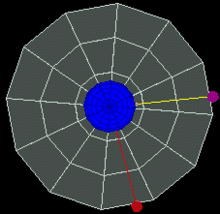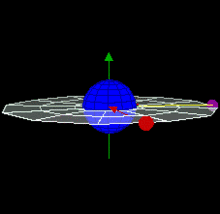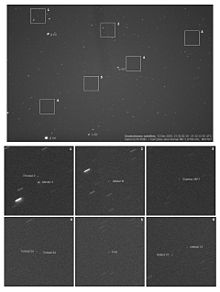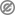# Geostationary orbit

﻿
Geostationary orbitGeostationary orbit.To an observer on the rotating Earth (fixed point on the Earth), the satellite appears stationary in the sky. A red satellite is also geostationary above its own point on Earth. Top Down ViewGeostationary orbit.To an observer on the rotating Earth (green dot on the blue sphere), the magenta satellite appears stationary in the sky. A red satellite is also geostationary above its own point on the blue sphereSide view of Geostationary 3D of 2 satellitesSide view of Geostationary 3D of 2 satellites of EarthA 5 x 6 degrees view of a part of the geostationary belt, showing several geostationary satellites. Those with inclination 0 degrees form a diagonal belt across the image: a few objects with small inclinations to the equator are visible above this line. Note how the satellites are pinpoint, while stars have created small trails due to the Earth's rotation.

A geostationary orbit (or Geostationary Earth Orbit - GEO) is a geosynchronous orbit directly above the Earth's equator (0° latitude), with a period equal to the Earth's rotational period and an orbital eccentricity of approximately zero. An object in a geostationary orbit appears motionless, at a fixed position in the sky, to ground observers. Communications satellites and weather satellites are often given geostationary orbits, so that the satellite antennas that communicate with them do not have to move to track them, but can be pointed permanently at the position in the sky where they stay. Due to the constant 0° latitude and circularity of geostationary orbits, satellites in GEO differ in location by longitude only.

The notion of a geosynchronous satellite for communication purposes was first published in 1928 (but not widely so) by Herman Potočnik. The idea of a geostationary orbit was first disseminated on a wide scale in a 1945 paper entitled "Extra-Terrestrial Relays — Can Rocket Stations Give Worldwide Radio Coverage?" by British science fiction writer Arthur C. Clarke, published in Wireless World magazine. The orbit, which Clarke first described as useful for broadcast and relay communications satellites, is sometimes called the Clarke Orbit. Similarly, the Clarke Belt is the part of space about 35,786 km (22,000 mi) above sea level, in the plane of the equator, where near-geostationary orbits may be implemented. The Clarke Orbit is about 265,000 km (165,000 mi) long.

Geostationary orbits are useful because they cause a satellite to appear stationary with respect to a fixed point on the rotating Earth, allowing a fixed antenna to maintain a link with the satellite. The satellite orbits in the direction of the Earth's rotation, at an altitude of 35,786 km (22,236 mi) above ground, producing an orbital period equal to the Earth's period of rotation, known as the sidereal day.

## Introduction

A geostationary transfer orbit is used to move a satellite from low Earth orbit (LEO) into a geostationary orbit.

A worldwide network of operational geostationary meteorological satellites is used to provide visible and infrared images of Earth's surface and atmosphere. These satellite systems include:

Most commercial communications satellites, broadcast satellites and SBAS satellites operate in geostationary orbits. (Russian television satellites have used elliptical Molniya and Tundra orbits due to the high latitudes of the receiving audience.) The first satellite placed into a geostationary orbit was the Syncom-3, launched by a Delta-D rocket in 1964.

A statite, a hypothetical satellite that uses a solar sail to modify its orbit, could theoretically hold itself in a "geostationary" orbit with different altitude and/or inclination from the "traditional" equatorial geostationary orbit.

## Derivation of geostationary altitude

In any circular orbit, the centripetal force required to maintain the orbit is provided by the gravitational force on the satellite. To calculate the geostationary orbit altitude, one begins with this equivalence, and uses the fact that the orbital period is one sidereal day.$\mathbf{F}_\text{c} = \mathbf{F}_\text{g}$

By Newton's second law of motion, we can replace the forces F with the mass m of the object multiplied by the acceleration felt by the object due to that force:$m \mathbf{a}_\text{c} = m \mathbf{g}$

We note that the mass of the satellite m appears on both sides — geostationary orbit is independent of the mass of the satellite. So calculating the altitude simplifies into calculating the point where the magnitudes of the centripetal acceleration required for orbital motion and the gravitational acceleration provided by Earth's gravity are equal.

The centripetal acceleration's magnitude is:$|\mathbf{a}_\text{c}| = \omega^2 r$

where ω is the angular speed, and r is the orbital radius as measured from the Earth's center of mass.

The magnitude of the gravitational acceleration is:$|\mathbf{g}| = \frac{G M}{r^2}$

where M is the mass of Earth, 5.9736 × 1024 kg, and G is the gravitational constant, 6.67428 ± 0.00067 × 10−11 m3 kg−1 s−2.

Equating the two accelerations gives:$r^3 = \frac{G M}{\omega^2} \to r = \sqrt{\frac{G M}{\omega^2}}$

The product GM is known with much greater precision than either factor alone; it is known as the geocentric gravitational constant μ = 398,600.4418 ± 0.0008 km3 s−2:$r = \sqrt{\frac\mu{\omega^2}}$

The angular speed ω is found by dividing the angle travelled in one revolution (360° = 2π rad) by the orbital period (the time it takes to make one full revolution: one sidereal day, or 86,164.09054 seconds). This gives:$\omega \approx \frac{2 \mathrm\pi~\mathrm{rad}} {86\,164~\mathrm{s}} \approx 7.2921 \times 10^{-5}~\mathrm{rad} / \mathrm{s}$

The resulting orbital radius is 42,164 kilometres (26,199 mi). Subtracting the Earth's equatorial radius, 6,378 kilometres (3,963 mi), gives the altitude of 35,786 kilometres (22,236 mi).

Orbital speed (how fast the satellite is moving through space) is calculated by multiplying the angular speed by the orbital radius:$v = \omega r \approx 3.0746~\mathrm{km}/\mathrm{s} \approx 11\,068~\mathrm{km}/\mathrm{h} \approx 6877.8~\mathrm{mph}\text{.}$

Now, by the same formula, let us find the geostationary-type orbit of an object in relation to Mars (this type of orbit above is referred to as an areostationary orbit if it is above Mars). The geocentric gravitational constant GM (which is μ) for Mars has the value of 42,828 km3s-2, and the known rotational period (T) of Mars is 88,642.66 seconds. Since ω = 2π/T, using the formula above, the value of ω is found to be approx 7.088218×10-5 s-1. Thus, r3 = 8.5243×1012 km3, whose cube root is 20,427 km; subtracting the equatorial radius of Mars (3396.2 km) we have 17,031 km.

## Practical limitations

A geostationary orbit can only be achieved at an altitude very close to 35,786 km (22,236 mi), and directly above the equator. This equates to an orbital velocity of 3.07 km/s (1.91 mi/s) or a period of 1,436 minutes, which equates to almost exactly one sidereal day or 23.934461223 hours. This makes sense considering that the satellite must be locked to the Earth's rotational period in order to have a stationary footprint on the ground. In practice, this means that all geostationary satellites have to exist on this ring, which poses problems for satellites that will be decommissioned at the end of their service lives (e.g., when they run out of thruster fuel). Such satellites will either continue to be used in inclined orbits (where the orbital track appears to follow a figure-eight loop centered on the equator), or else be elevated to a "graveyard" disposal orbit.

A combination of lunar gravity, solar gravity, and the flattening of the Earth at its poles is causing a precession motion of the orbit plane of any geostationary object with a period of about 53 years and an initial inclination gradient of about 0.85 degrees per year, achieving a maximum inclination of 15 degrees after 26.5 years. To correct for this orbital perturbation, regular orbital stationkeeping maneuvers are necessary, amounting to a delta-v of approximately 50 m/s per year.

The second effect to be taken into account is the longitude drift, caused by the asymmetry of the earth - the equator is slightly elliptical. There are two stable (at 75.3°E, and at 104.7°W) and two unstable (at 165.3°E, and at 14.7°W) equilibrium points. Any geostationary object placed between the equilibrium points would (without any action) be slowly accelerated towards the stable equilibrium position, causing a periodic longitude variation. The correction of this effect requires orbit control maneuvers with a maximum delta-v of about 2 m/s per year, depending on the desired longitude.

In the absence of servicing missions from the Earth, the consumption of thruster propellant for station-keeping places a limitation on the lifetime of the satellite.

### Communications

Satellites in geostationary orbits are far enough away from Earth that communication latency becomes very high — about a quarter of a second for a one-way trip from one ground based transmitter to another via the geostationary satellite; close to half a second for round-trip communication between two earth stations.

For example, for ground stations at latitudes of φ=±45° on the same meridian as the satellite, the one-way delay can be computed by using the cosine rule, given the above derived geostationary orbital radius r, the Earth's radius R and the speed of light c, as$2 \frac {\sqrt{R^2+r^2-2 R r \cos\varphi}} c \approx253\,\mathrm{ms}$

This presents problems for latency-sensitive applications such as voice communication or online gaming.

## Orbit allocation

Satellites in geostationary orbit must all occupy a single ring above the equator. The requirement to space these satellites apart to avoid harmful radio-frequency interference during operations means that there are a limited number of orbital "slots" available, thus only a limited number of satellites can be operated in geostationary orbit. This has led to conflict between different countries wishing access to the same orbital slots (countries at the same longitude but differing latitudes) and radio frequencies. These disputes are addressed through the International Telecommunication Union's allocation mechanism. Countries located at the Earth's equator have also asserted their legal claim to control the use of space above their territory.

## Notes and references

1. ^ Noordung, Hermann; et al. (1995) . The Problem With Space Travel. Translation from original German. DIANE Publishing. pp. 72. ISBN 978-0788118494.
2. ^ "Extra-Terrestrial Relays — Can Rocket Stations Give Worldwide Radio Coverage?". Arthur C. Clark. October 1945. Retrieved 2009-03-04.
3. ^ "Basics of Space Flight Section 1 Part 5, Geostationary Orbits". NASA. Retrieved 2009-06-21.
4. ^ In the small body approximation, the geostationary orbit is independent of the satellite's mass. For satellites having a mass less than M μerr/μ≈1015 kg, that is, over a billion times that of the ISS, the error due to the approximation is smaller than the error on the universal geocentric gravitational constant (and thus negligible).
5. ^ Edited by P. Kenneth Seidelmann, "Explanatory Supplement to the Astronomical Almanac", University Science Books,1992, pp. 700
6. ^ The Teledesic Network: Using Low-Earth-Orbit Satellites to Provide Broadband, Wireless, Real-Time Internet Access Worldwide
7. ^ http://www.itu.int/ITU-R/conferences/seminars/mexico-2001/docs/06-procedure-mechanism.doc
8. ^ Oduntan, Gbenga.   Hertfordshire Law Journal, 1(2), p. 75.This article incorporates public domain material from the General Services Administration document "Federal Standard 1037C" (in support of MIL-STD-188).

Wikimedia Foundation. 2010.

### Look at other dictionaries:

• geostationary orbit —       a circular orbit 35,785 km (22,236 miles) above Earth s (Earth) Equator in which a satellite s (satellite) orbital period is equal to Earth s rotation period of 23 hours and 56 minutes. A spacecraft in this orbit appears to an observer on… …   Universalium

• geostationary orbit — noun a geosynchronous orbit that is fixed with respect to a position on the Earth • Hypernyms: ↑geosynchronous orbit * * * geostationary orbit, the orbit of a synchronous satellite; an orbit in which an artificial satellite moves at the same rate …   Useful english dictionary

• Geostationary Orbit —    A spaceship or satellite in orbit 35,900 kilometers above the equator with an orbital period of 24 hours. This orbit keeps the object over a specific Earth location at all times. This type of orbit is used most often with communication… …   The writer's dictionary of science fiction, fantasy, horror and mythology

• geostationary orbit —   an orbit path that keeps a satellite over the exact same point on the earth surface at all times …   Geography glossary

• geostationary orbit — /dʒioʊˌsteɪʃənri ˈɔbət/ (say jeeoh.stayshuhnree awbuht) noun → synchronous equatorial orbit …   Australian English dictionary

• Geostationary transfer orbit — A Geosynchronous Transfer Orbit or Geostationary Transfer Orbit (GTO) is a Hohmann transfer orbit around the Earth between a low Earth orbit (LEO) and a geosynchronous orbit (GEO). It is an ellipse where the perigee is a point on a LEO and the… …   Wikipedia

• Geostationary ring — The geostationary ring is a volume segment around the geostationary orbit defined by variations in altitude and declination that can occur for uncontrolled objects left in the geostationary orbit.The geostationary orbit is subject to orbit… …   Wikipedia

• Geostationary Operational Environmental Satellite — The Geostationary Operational Environmental Satellite (or GOES) program is a key element in United States National Weather Service (NWS) operations. GOES weather imagery and quantitative sounding data are a continuous and reliable stream of… …   Wikipedia

• geostationary — /jee oh stay sheuh ner ee/, adj. of or pertaining to a satellite traveling in an orbit 22,300 miles (35,900 km) above the earth s equator: at this altitude, the satellite s period of rotation, 24 hours, matches the earth s and the satellite… …   Universalium

• geostationary —    Also known as geosynchronous. The type of orbit required to keep a communications satellite in a fixed position relative to the earth.    The satellite s angular rate and direction of rotation are matched to those of the earth, and the… …   Dictionary of networking

We are using cookies for the best presentation of our site. Continuing to use this site, you agree with this.